# Matchings and minimum degree

A Tale of Two Halls

(Philip) Hall’s theorem.  Let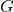be a bipartite graph on vertex classes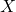,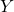.  Suppose that,  for every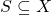,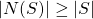.  Then there is a matching fromto.

This is traditionally called Hall’s marriage theorem.  The picture is that the people inare all prepared to marry some subset of the people in.  If some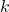people inare only prepared to marry into some set of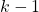people, then we have a problem; but this is the only problem we might have.  There is no room in this picture for the preferences of people in.

Proof. Suppose first that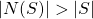for all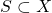.  Then we can match any element ofarbitrarily to a neighbour inand obtain a smaller graph on which Hall’s condition holds, so are done by induction.

Otherwise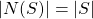for some.  By induction there is a matching from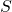to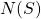.  Let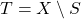.  Then for any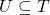we have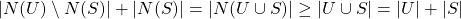hence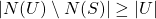and Hall’s condition holds on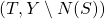.  So by induction there is a matching from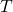to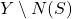, which together with the matching fromtois a matching fromto.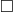Corollary 1.  Every-regular bipartite graph has a perfect matching.

Proof. Counted with multiplicity,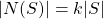.  But each element ofis hit at mosttimes, so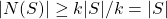in the conventional sense.Corollary 2. Letbe a spanning subgraph of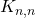with minimum degree at least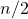.  Thenhas a perfect matching.

This is very slightly more subtle.

Proof. If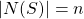there is nothing to check.  Otherwise there is a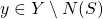.  Then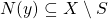and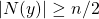, so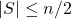.  But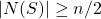for every.Schrijver proved that a-regular bipartite graph with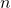vertices in each class in fact has at least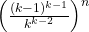perfect matchings.  For minimum degreewe have the following.

(Marshall) Hall’s theorem.  If each vertex ofhas degree at leastand there is at least one perfect matching then there are at least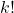.

This turns out to be easy if we were paying attention during the proof of (Philip) Hall’s theorem.

Proof. By (Philip) Hall’s theorem the existence of a perfect matching means that (Philip) Hall’s condition holds.  Choose a minimalon which it is tight.  Fix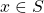and match it arbitrarily to a neighbour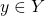.  (Philip) Hall’s condition still holds on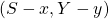and the minimum degree on this subgraph is at least, so by induction we can find at least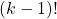perfect matchings.  Since there were at leastchoices for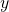we have at leastperfect matchings fromto.  These extend to perfect matchings fromtoas in the proof of (Philip) Hall’s theorem.Thanks to Gjergji Zaimi for pointing me to (Marshall) Hall’s paper via MathOverflow.